Question

# A satellite with mass 898 kg is in a circular orbit with an orbital speed of...

A satellite with mass 898 kg is in a circular orbit with an orbital speed of 5840 m/s around the earth.

a) What is the new orbital speed after friction from the earth's upper atmosphere has done −7.5×10^9 J of work on the satellite? Express your answer with the appropriate units.

b)  Does the speed increase or decrease?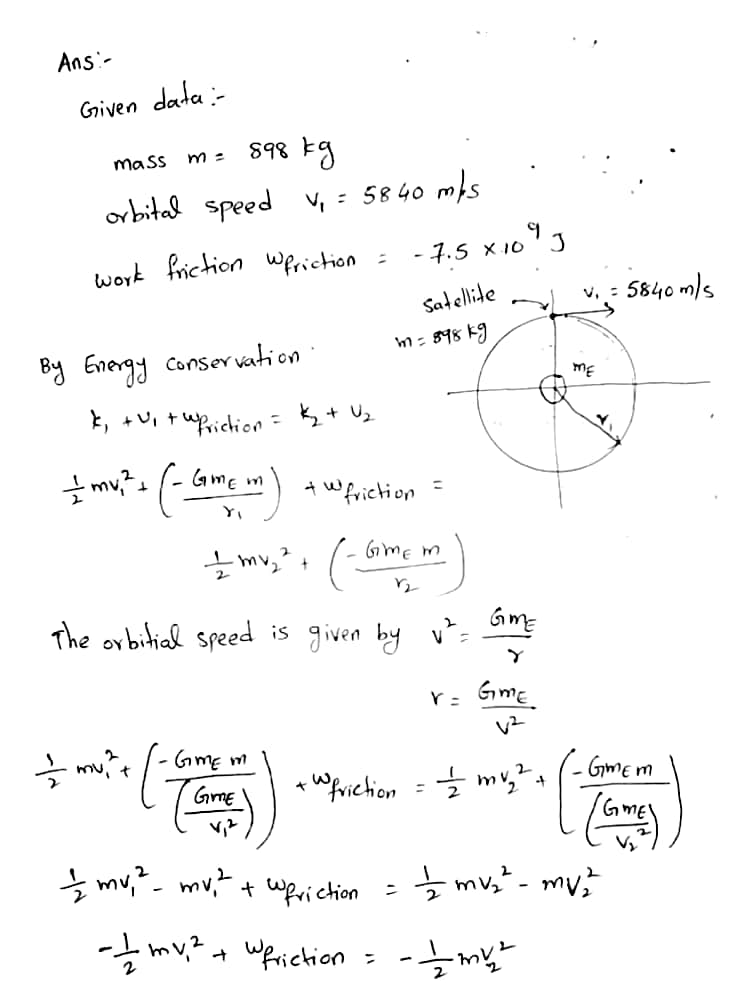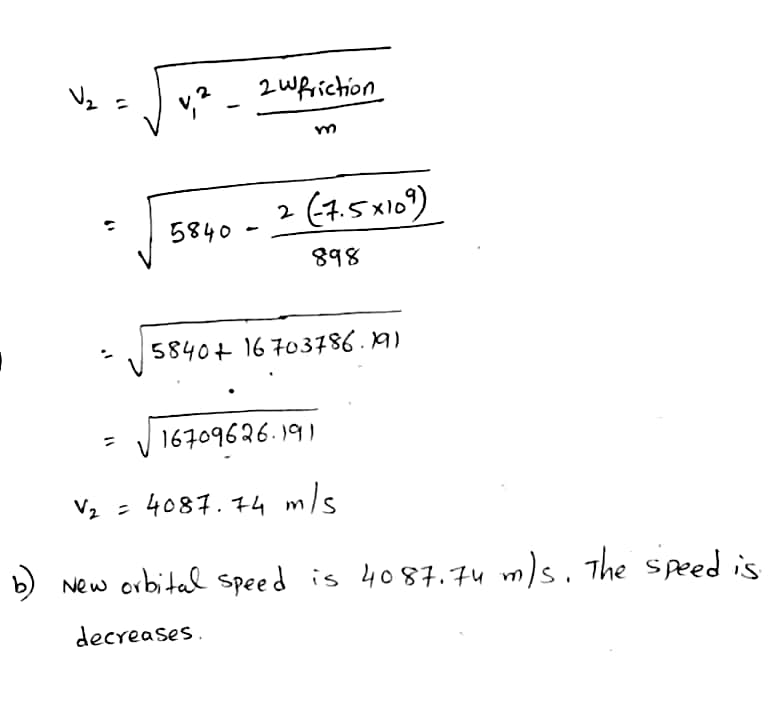#### Earn Coins

Coins can be redeemed for fabulous gifts.

Similar Homework Help Questions
• ### A satellite with mass 748 kg is in a circular orbit with an orbital speed of...

A satellite with mass 748 kg is in a circular orbit with an orbital speed of 9040 m/s around the earth. What is the new orbital speed after friction from the earth's upper atmosphere has done -7.5×109 J of work on the satellite? Express your answer with the appropriate units.

• ### A satellite with mass 948 kg is in a circular orbit with an orbital speed of 5840 m/s around the earth.

What is the new orbital speed after friction from the earth's upper atmosphere has done −7.5×10^9J of work on the satellite?Express your answer with the appropriate units.

• ### please refer to picture A satellite is in a circular orbit around the Earth. To increase...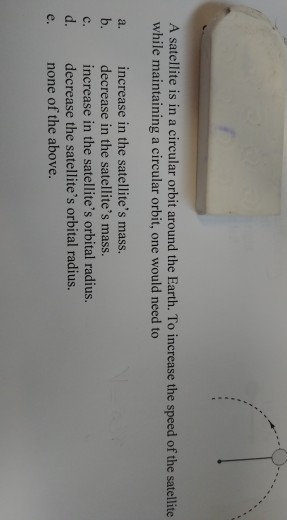please refer to picture A satellite is in a circular orbit around the Earth. To increase the speed of the satellite while maintaining a circular orbit, one would need to a. b. c. d. increase in the satellite's mass. decrease in the satellite's mass. increase in the satellite's orbital radius. decrease the satellite's orbital radius. none of the above.

• ### 4. A 1000-kg satellite in circular orbit around the Earth is moving at a speed of...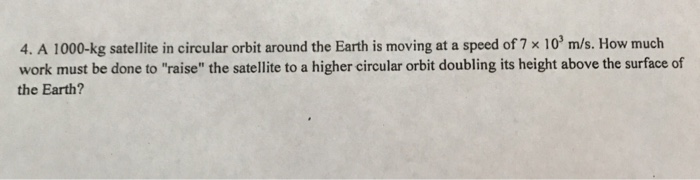4. A 1000-kg satellite in circular orbit around the Earth is moving at a speed of 7 x 10 m/s. How much work must be done to "raise" the satellite to a higher circular orbit doubling its height above the surface of the Earth?

• ### A 544-kg satellite is in a circular orbit about Earth at a height above Earth equal...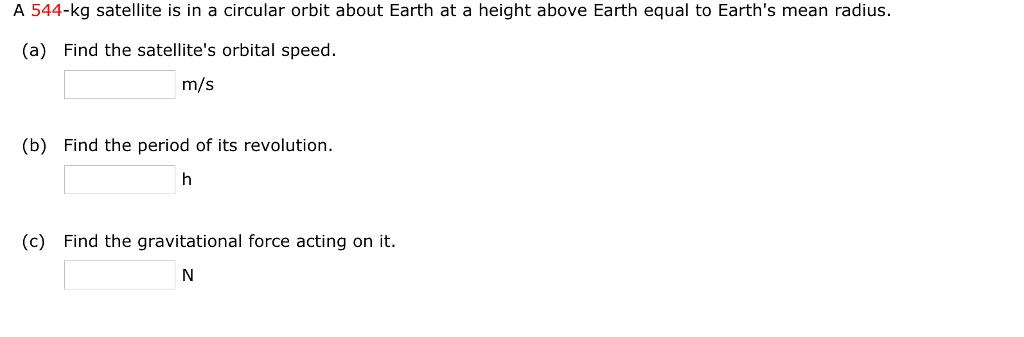A 544-kg satellite is in a circular orbit about Earth at a height above Earth equal to Earth's mean radius. (a) Find the satellite's orbital speed. m/s (b) Find the period of its revolution. (c) Find the gravitational force acting on it A satellite of Mars, called Phobos, has an orbital radius of 9.4 x 106 m and a period of 2.8 104 s. Assuming the orbit is circular, determine the mass of Mars. x 10 s. Assuming kg

• ### What speed must a satellite have if it is to move in a circular orbit of...

What speed must a satellite have if it is to move in a circular orbit of 610 km above the surface of the Earth? (The Earth's radius is 6400 km and the Earth's mass is 5.98*1024 kg.)

• ### A 180 kg satellite is orbiting on a circular orbit 6355 km above the Earth's surface. Determine the speed of the satell...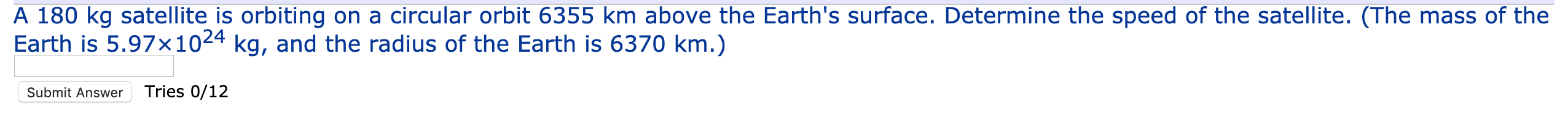A 180 kg satellite is orbiting on a circular orbit 6355 km above the Earth's surface. Determine the speed of the satellite. (The mass of the Earth is 5.97x1024 kg, and the radius of the Earth is 6370 km.) Submit Answer Tries 0/12

• ### Two satellites are in circular orbits around the earth. The orbit for satellite A is at...

Two satellites are in circular orbits around the earth. The orbit for satellite A is at a height of 364 km above the earth's surface, while that for satellite B is at a height of 880. km. Find the orbital speed for satellite A and satellite B. (a) VA = (b) VB =

• ### Consider a satellite of mass m moving in a circular orbit around the Earth at a...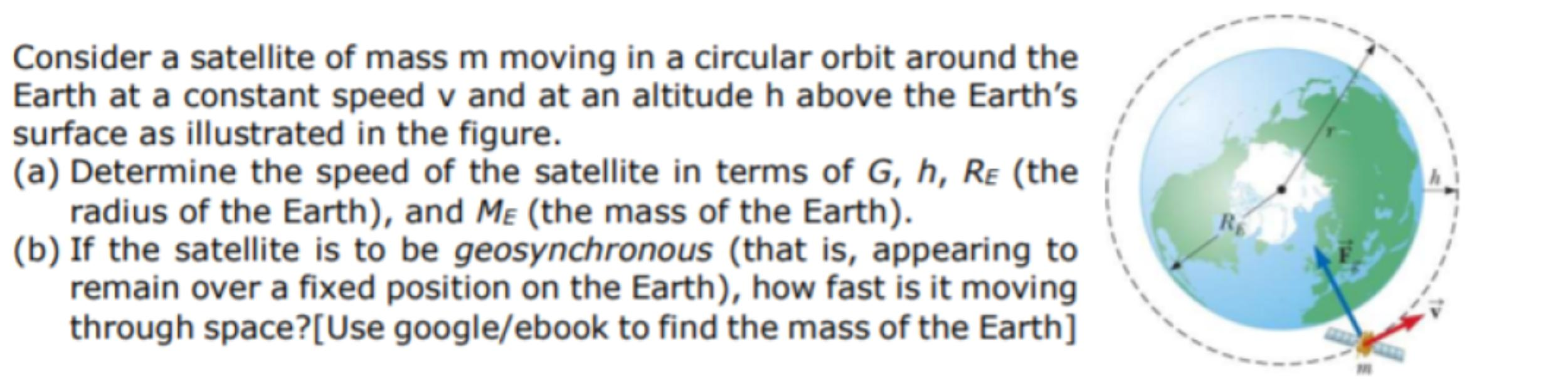Consider a satellite of mass m moving in a circular orbit around the Earth at a constant speed v and at an altitude h above the Earth's surface as illustrated in the figure. (a) Determine the speed of the satellite in terms of G, h, Re (the radius of the Earth), and Me (the mass of the Earth). (b) If the satellite is to be geosynchronous (that is, appearing to remain over a fixed position on the Earth), how fast...

• ### What is the orbital speed of a satelite in a circular orbit at 8, 129 kilometers...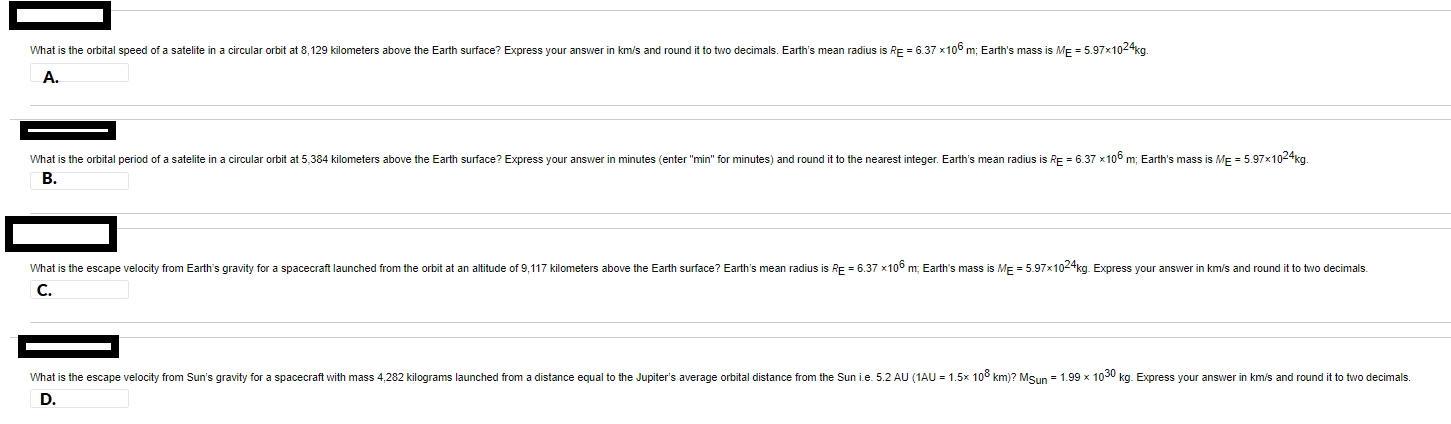What is the orbital speed of a satelite in a circular orbit at 8, 129 kilometers above the Earth surface? Express your answer in km/s and round it to two decimals. Earth's mean radius is RE = 6.37 x100 m; Earth's mass is ME = 5.97x104kg. A What is the orbital period of a satelite in a circular orbit at 5,384 kilometers above the Earth surface? Express your answer in minutes (enter "min" for minutes) and round it to the...